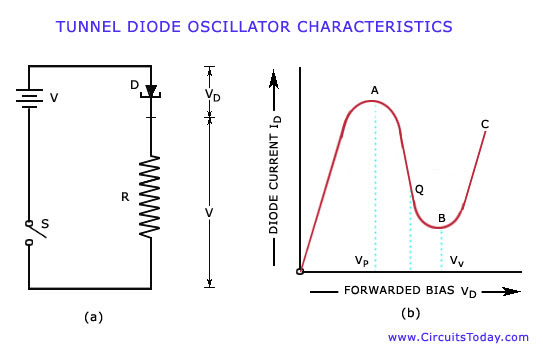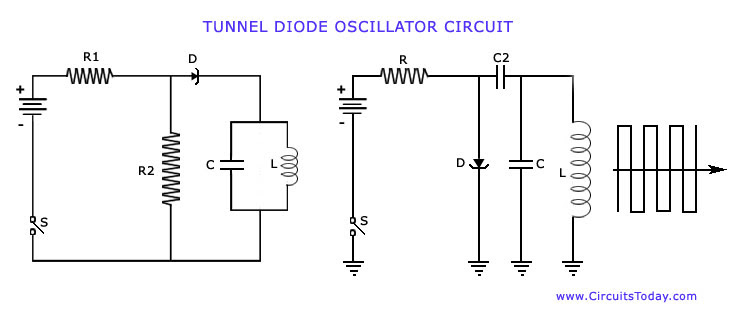## Audio

[Audio][slideshow]

In this article, the working of a negative resistance oscillator is explained along with its types-dynatron and tunnel diode oscillator. The tunnel diode oscillator characteristics and circuit is also explained in detail.

Related Articles

VOLTAGE CONTROLLED OSCILLATOR (VCO)

SWEEP-FREQUENCY GENERATOR

BEAT FREQUENCY OSCILLATOR (BFO)

WIEN BRIDGE OSCILLATOR

PHASE SHIFT OSCILLATOR

TRANSISTOR PHASE SHIFT OSCILLATOR

Negative resistance oscillators make use of negative resistance elements such as tetrodes, tunnel diodes, uni junction transistors etc. There are two types of negative resistance oscillators, which are commonly used for high frequency generation. These are dynatron and tunnel diode oscil­lators.

Dynatron operates in the negative resistance region of the characteristics of a tetrode, which is coupled to an L-C tank circuit.

Tunnel diode oscillator makes use of a tunnel diode for producing oscillations.Now consider a circuit as shown in the figure (a) shown above, where D is a tunnel diode, R is an external resistance, V is the battery voltage, VD is voltage drop across diode and VR is voltage drop across resistance R. The value of R is carefully selected and its value depends on the bias of the diode D in the negative resistance region AB. From the graph we can see that the quiescent point Q is almost at the center of the characteristic curve AB.

The resistance R and the diode resistance are kept in series and this determines the rise in value of the current when the switch S is closed. According to the ratio of their resistances, the applied voltage V divides across D and R. However, as diode voltage VD become more than Vp (point A), the diode is driven into the negative resistance region and its resistance starts increasing. So VD increases until it becomes equal to the valley voltage Vv (point B). At this point further increase in VD drives the diode into the positive resistance re­gion BC [figure (b)]. Now increase in current causes increase in VR and de­crease in VD, thereby bringing the diode back into the negative resistance region. This reduction in diode voltage VD causes an increase in circuit current till point A is reached when VD equals Vp. Thus the circuit will continue to oscillate back and forth through the negative resistance region, that is between points A and B on its characteristic. Its output across external resistance R is sinusoidal. A practical circuit of a tunnel diode oscillator in two slightly dif­ferent ways is shown in the figure shown below. Here R2 sets the proper bias level for the diode whereas Rx in parallel with the L-C tank circuit sets proper current level for it. The capacitor Cc is the coupling capacitor. As the switch is closed, the tunnel diode is set into oscillations whose frequency is equal to the resonant frequency of the tank circuit.The tunnel diode operates very fast and it is possible to make tunnel diode oscillators which operate in the microwave frequency region.

[Lights][grids]